# Complex models in ecology: challenges and solutions

May. 27, 2013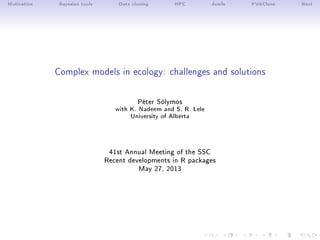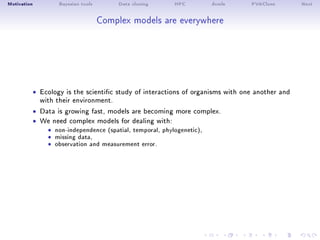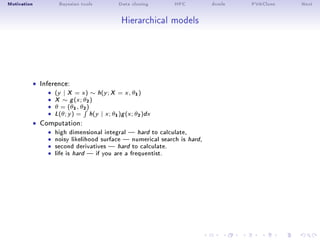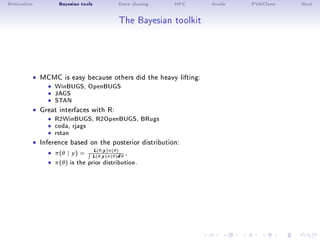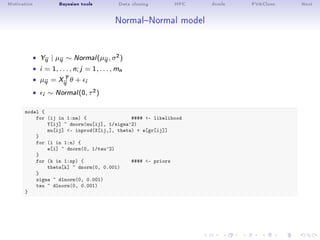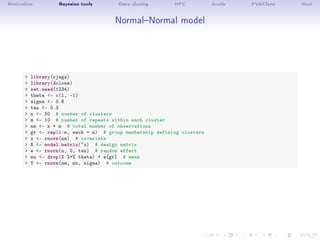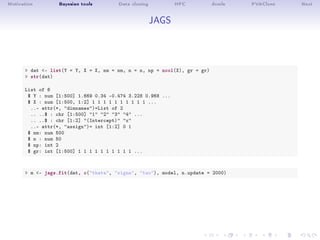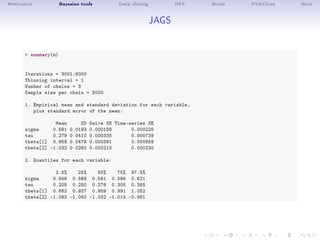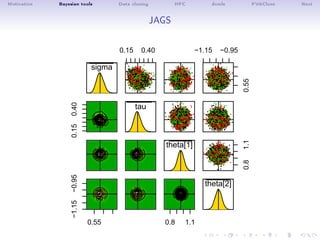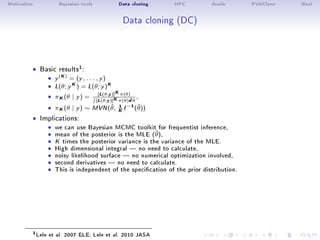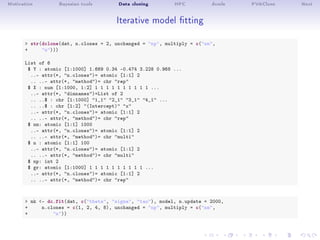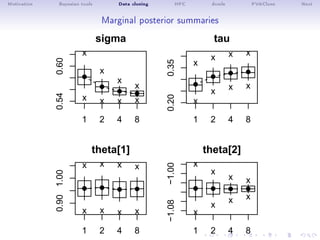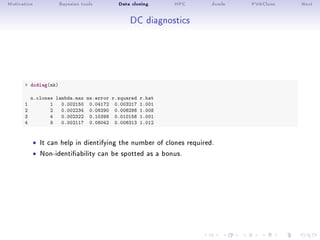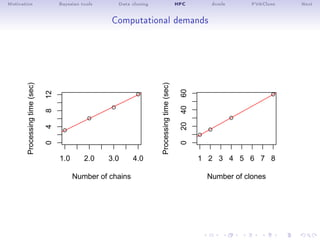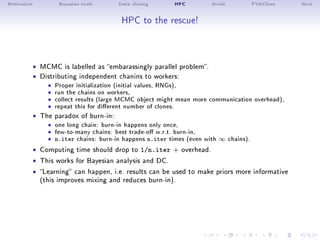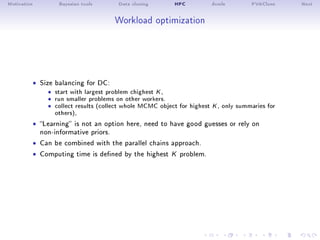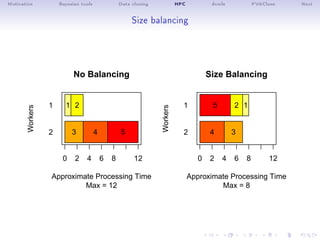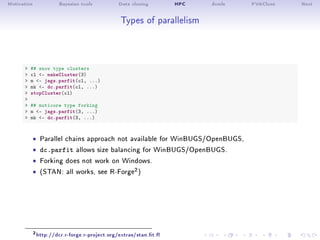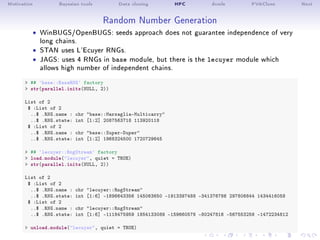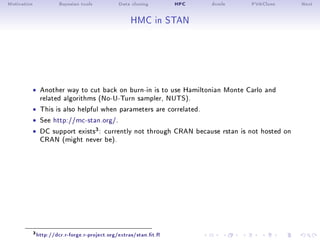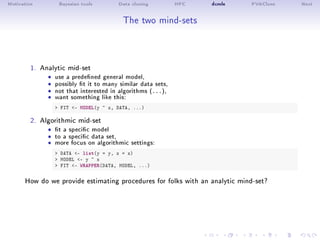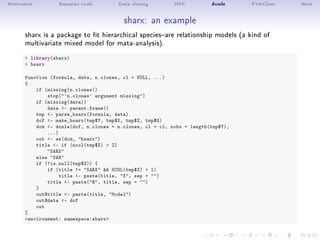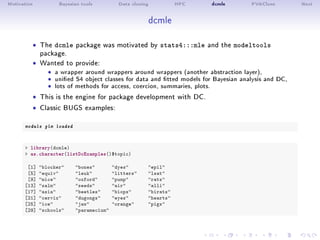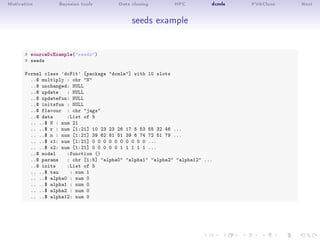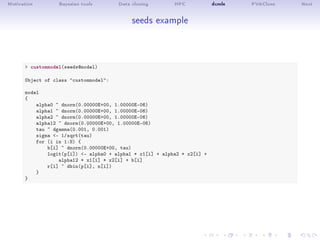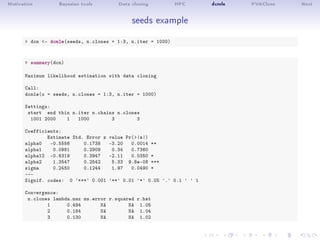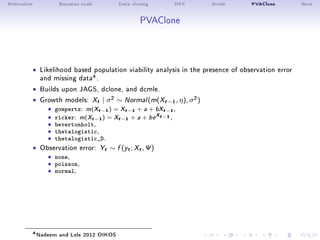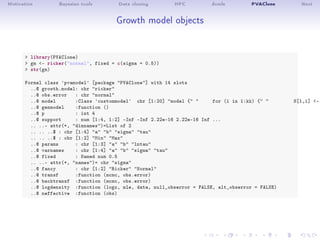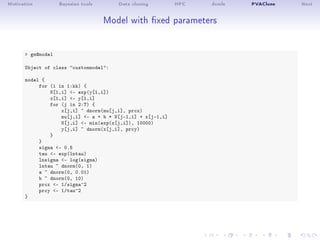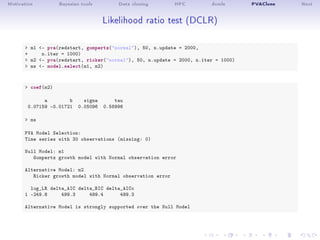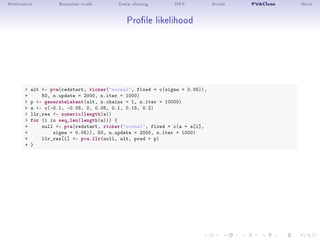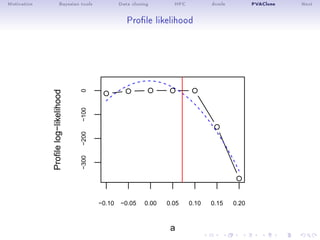1 of 33

### Complex models in ecology: challenges and solutions

1. Motivation Bayesian tools Data cloning HPC dcmle PVAClone Next Complex models in ecology: challenges and solutions Péter Sólymos with K. Nadeem and S. R. Lele University of Alberta 41st Annual Meeting of the SSC Recent developments in R packages May 27, 2013
2. Motivation Bayesian tools Data cloning HPC dcmle PVAClone Next Complex models are everywhere ˆ Ecology is the scientic study of interactions of organisms with one another and with their environment. ˆ Data is growing fast, models are becoming more complex. ˆ We need complex models for dealing with: ˆ non-independence (spatial, temporal, phylogenetic),ˆ missing data,ˆ observation and measurement error.
3. Motivation Bayesian tools Data cloning HPC dcmle PVAClone Next Hierarchical models ˆ Inference: ˆ (y | X = x) ∼ h(y ; X = x, θ1) ˆ X ∼ g (x; θ2) ˆ θ = (θ1, θ2) ˆ L(θ; y ) = h(y | x; θ1)g (x; θ2)dx ˆ Computation: ˆ high dimensional integral hard to calculate,ˆ noisy likelihood surface numerical search is hard,ˆ second derivatives hard to calculate.ˆ life is hard if you are a frequentist.
4. Motivation Bayesian tools Data cloning HPC dcmle PVAClone Next The Bayesian toolkit ˆ MCMC is easy because others did the heavy lifting: ˆ WinBUGS, OpenBUGSˆ JAGSˆ STAN ˆ Great interfaces with R: ˆ R2WinBUGS, R2OpenBUGS, BRugsˆ coda, rjagsˆ rstan ˆ Inference based on the posterior distribution: ˆ π(θ | y ) = L(θ;y)π(θ) L(θ;y)π(θ)dθ , ˆ π(θ) is the prior distribution.
5. Motivation Bayesian tools Data cloning HPC dcmle PVAClone Next NormalNormal model ˆ Yij | µij ∼ Normal(µij, σ2) ˆ i = 1, . . . , n; j = 1, . . . , mn ˆ µij = XT ij θ + i ˆ i ∼ Normal(0, τ2) model { for (ij in 1:nm) { #### - likelihood Y[ij] ~ dnorm(mu[ij], 1/sigma^2) mu[ij] - inprod(X[ij,], theta) + e[gr[ij]] } for (i in 1:n) { e[i] ~ dnorm(0, 1/tau^2) } for (k in 1:np) { #### - priors theta[k] ~ dnorm(0, 0.001) } sigma ~ dlnorm(0, 0.001) tau ~ dlnorm(0, 0.001) }
6. Motivation Bayesian tools Data cloning HPC dcmle PVAClone Next NormalNormal model library(rjags) library(dclone) set.seed(1234) theta - c(1, -1) sigma - 0.6 tau - 0.3 n - 50 # number of clusters m - 10 # number of repeats within each cluster nm - n * m # total number of observations gr - rep(1:n, each = m) # group membership defining clusters x - rnorm(nm) # covariate X - model.matrix(~x) # design matrix e - rnorm(n, 0, tau) # random effect mu - drop(X %*% theta) + e[gr] # mean Y - rnorm(nm, mu, sigma) # outcome
7. Motivation Bayesian tools Data cloning HPC dcmle PVAClone Next JAGS dat - list(Y = Y, X = X, nm = nm, n = n, np = ncol(X), gr = gr) str(dat) List of 6 \$ Y : num [1:500] 1.669 0.34 -0.474 3.228 0.968 ... \$ X : num [1:500, 1:2] 1 1 1 1 1 1 1 1 1 1 ... ..- attr(*, dimnames)=List of 2 .. ..\$ : chr [1:500] 1 2 3 4 ... .. ..\$ : chr [1:2] (Intercept) x ..- attr(*, assign)= int [1:2] 0 1 \$ nm: num 500 \$ n : num 50 \$ np: int 2 \$ gr: int [1:500] 1 1 1 1 1 1 1 1 1 1 ... m - jags.fit(dat, c(theta, sigma, tau), model, n.update = 2000)
8. Motivation Bayesian tools Data cloning HPC dcmle PVAClone Next JAGS summary(m) Iterations = 3001:8000 Thinning interval = 1 Number of chains = 3 Sample size per chain = 5000 1. Empirical mean and standard deviation for each variable, plus standard error of the mean: Mean SD Naive SE Time-series SE sigma 0.581 0.0193 0.000158 0.000225 tau 0.279 0.0410 0.000335 0.000739 theta 0.959 0.0479 0.000391 0.000959 theta -1.032 0.0260 0.000213 0.000230 2. Quantiles for each variable: 2.5% 25% 50% 75% 97.5% sigma 0.546 0.568 0.581 0.594 0.621 tau 0.205 0.250 0.276 0.305 0.365 theta 0.862 0.927 0.959 0.991 1.052 theta -1.083 -1.050 -1.032 -1.014 -0.981
9. Motivation Bayesian tools Data cloning HPC dcmle PVAClone Next JAGS sigma 0.15 0.40 −1.15 −0.95 0.55 0.150.40 20 40 tau 20 10 theta 0.81.1 0.55 −1.15−0.95 50 20 0.8 1.1 10 theta
10. Motivation Bayesian tools Data cloning HPC dcmle PVAClone Next Data cloning (DC) ˆ Basic results1: ˆ y (K) = (y , . . . , y ) ˆ L(θ; y K) = L(θ; y )K ˆ πK(θ | y ) = [L(θ;y)]Kπ(θ) [L(θ;y)]Kπ(θ)dθ , ˆ πK(θ | y ) ∼ MVN(ˆθ, 1 K I −1(ˆθ)) ˆ Implications: ˆ we can use Bayesian MCMC toolkit for frequentist inference,ˆ mean of the posterior is the MLE (ˆθ),ˆ K times the posterior variance is the variance of the MLE.ˆ High dimensional integral no need to calculate,ˆ noisy likelihood surface no numerical optimization involved,ˆ second derivatives no need to calculate.ˆ This is independent of the specication of the prior distribution. 1Lele et al. 2007 ELE; Lele et al. 2010 JASA
11. Motivation Bayesian tools Data cloning HPC dcmle PVAClone Next Iterative model tting str(dclone(dat, n.clones = 2, unchanged = np, multiply = c(nm, + n))) List of 6 \$ Y : atomic [1:1000] 1.669 0.34 -0.474 3.228 0.968 ... ..- attr(*, n.clones)= atomic [1:1] 2 .. ..- attr(*, method)= chr rep \$ X : num [1:1000, 1:2] 1 1 1 1 1 1 1 1 1 1 ... ..- attr(*, dimnames)=List of 2 .. ..\$ : chr [1:1000] 1_1 2_1 3_1 4_1 ... .. ..\$ : chr [1:2] (Intercept) x ..- attr(*, n.clones)= atomic [1:1] 2 .. ..- attr(*, method)= chr rep \$ nm: atomic [1:1] 1000 ..- attr(*, n.clones)= atomic [1:1] 2 .. ..- attr(*, method)= chr multi \$ n : atomic [1:1] 100 ..- attr(*, n.clones)= atomic [1:1] 2 .. ..- attr(*, method)= chr multi \$ np: int 2 \$ gr: atomic [1:1000] 1 1 1 1 1 1 1 1 1 1 ... ..- attr(*, n.clones)= atomic [1:1] 2 .. ..- attr(*, method)= chr rep mk - dc.fit(dat, c(theta, sigma, tau), model, n.update = 2000, + n.clones = c(1, 2, 4, 8), unchanged = np, multiply = c(nm, + n))
12. Motivation Bayesian tools Data cloning HPC dcmle PVAClone Next Marginal posterior summaries sigma Number of clones 1 2 4 8 0.540.60 x x x x x x x x tau Number of clones Estimate 1 2 4 8 0.200.35 x x x x x x x x theta 1 2 4 8 0.901.00 x x x x x x x x thetaEstimate 1 2 4 8 −1.08−1.00 x x x x x x x x
13. Motivation Bayesian tools Data cloning HPC dcmle PVAClone Next DC diagnostics dcdiag(mk) n.clones lambda.max ms.error r.squared r.hat 1 1 0.002150 0.04172 0.003217 1.001 2 2 0.002234 0.08290 0.006286 1.008 3 4 0.002322 0.10398 0.010156 1.001 4 8 0.002117 0.08042 0.006313 1.012 ˆ It can help in dientifying the number of clones required. ˆ Non-identiability can be spotted as a bonus.
14. Motivation Bayesian tools Data cloning HPC dcmle PVAClone Next Computational demands 1.0 2.0 3.0 4.0 04812 Number of chains Processingtime(sec) 1 2 3 4 5 6 7 8 0204060 Number of clones Processingtime(sec)
15. Motivation Bayesian tools Data cloning HPC dcmle PVAClone Next HPC to the rescue! ˆ MCMC is labelled as embarassingly parallel problem. ˆ Distributing independent chanins to workers: ˆ Proper initialization (initial values, RNGs),ˆ run the chains on workers,ˆ collect results (large MCMC object might mean more communication overhead),ˆ repeat this for dierent number of clones. ˆ The paradox of burn-in: ˆ one long chain: burn-in happens only once,ˆ few-to-many chains: best trade-o w.r.t. burn-in,ˆ n.iter chains: burn-in happens n.iter times (even with ∞ chains). ˆ Computing time should drop to 1/n.iter + overhead. ˆ This works for Bayesian analysis and DC. ˆ Learning can happen, i.e. results can be used to make priors more informative (this improves mixing and reduces burn-in).
16. Motivation Bayesian tools Data cloning HPC dcmle PVAClone Next Workload optimization ˆ Size balancing for DC: ˆ start with largest problem chighest K ,ˆ run smaller problems on other workers.ˆ collect results (collect whole MCMC object for highest K , only summaries for others), ˆ Learning is not an option here, need to have good guesses or rely on non-informative priors. ˆ Can be combined with the parallel chains approach. ˆ Computing time is dened by the highest K problem.
17. Motivation Bayesian tools Data cloning HPC dcmle PVAClone Next Size balancing 0 2 4 6 8 12 2 1 No Balancing Max = 12 Approximate Processing Time Workers 1 2 3 4 5 0 2 4 6 8 12 2 1 Size Balancing Max = 8 Approximate Processing Time Workers 5 2 1 4 3
18. Motivation Bayesian tools Data cloning HPC dcmle PVAClone Next Types of parallelism ## snow type clusters cl - makeCluster(3) m - jags.parfit(cl, ...) mk - dc.parfit(cl, ...) stopCluster(cl) ## muticore type forking m - jags.parfit(3, ...) mk - dc.parfit(3, ...) ˆ Parallel chains approach not available for WinBUGS/OpenBUGS, ˆ dc.parfit allows size balancing for WinBUGS/OpenBUGS. ˆ Forking does not work on Windows. ˆ (STAN: all works, see R-Forge2) 2http://dcr.r-forge.r-project.org/extras/stan.t.R
19. Motivation Bayesian tools Data cloning HPC dcmle PVAClone Next Random Number Generation ˆ WinBUGS/OpenBUGS: seeds approach does not guarantee independence of very long chains. ˆ STAN uses L'Ecuyer RNGs. ˆ JAGS: uses 4 RNGs in base module, but there is the lecuyer module which allows high number of independent chains. ## 'base::BaseRNG' factory str(parallel.inits(NULL, 2)) List of 2 \$ :List of 2 ..\$ .RNG.name : chr base::Marsaglia-Multicarry ..\$ .RNG.state: int [1:2] 2087563718 113920118 \$ :List of 2 ..\$ .RNG.name : chr base::Super-Duper ..\$ .RNG.state: int [1:2] 1968324500 1720729645 ## 'lecuyer::RngStream' factory load.module(lecuyer, quiet = TRUE) str(parallel.inits(NULL, 2)) List of 2 \$ :List of 2 ..\$ .RNG.name : chr lecuyer::RngStream ..\$ .RNG.state: int [1:6] -1896643356 145063650 -1913397488 -341376786 297806844 1434416058 \$ :List of 2 ..\$ .RNG.name : chr lecuyer::RngStream ..\$ .RNG.state: int [1:6] -1118475959 1854133089 -159660578 -80247816 -567553258 -1472234812 unload.module(lecuyer, quiet = TRUE)
20. Motivation Bayesian tools Data cloning HPC dcmle PVAClone Next HMC in STAN ˆ Another way to cut back on burn-in is to use Hamiltonian Monte Carlo and related algorithms (No-U-Turn sampler, NUTS). ˆ This is also helpful when parameters are correlated. ˆ See http://mc-stan.org/. ˆ DC support exists3: currently not through CRAN because rstan is not hosted on CRAN (might never be). 3http://dcr.r-forge.r-project.org/extras/stan.t.R
21. Motivation Bayesian tools Data cloning HPC dcmle PVAClone Next The two mind-sets 1. Analytic mid-set ˆ use a predened general model,ˆ possibly t it to many similar data sets,ˆ not that interested in algorithms (...),ˆ want something like this: FIT - MODEL(y ~ x, DATA, ...) 2. Algorithmic mid-set ˆ t a specic modelˆ to a specic data set,ˆ more focus on algorithmic settings: DATA - list(y = y, x = x) MODEL - y ~ x FIT - WRAPPER(DATA, MODEL, ...) How do we provide estimating procedures for folks with an analytic mind-set?
22. Motivation Bayesian tools Data cloning HPC dcmle PVAClone Next sharx: an example sharx is a package to t hierarchical speciesare relationship models (a kind of multivariate mixed model for mata-analysis). library(sharx) hsarx function (formula, data, n.clones, cl = NULL, ...) { if (missing(n.clones)) stop('n.clones' argument missing) if (missing(data)) data - parent.frame() tmp - parse_hsarx(formula, data) dcf - make_hsarx(tmp\$Y, tmp\$X, tmp\$Z, tmp\$G) dcm - dcmle(dcf, n.clones = n.clones, cl = cl, nobs = length(tmp\$Y), ...) out - as(dcm, hsarx) title - if (ncol(tmp\$X) 2) SARX else SAR if (!is.null(tmp\$Z)) { if (title != SARX NCOL(tmp\$Z) 1) title - paste(title, X, sep = ) title - paste(H, title, sep = ) } out@title - paste(title, Model) out@data - dcf out } environment: namespace:sharx
23. Motivation Bayesian tools Data cloning HPC dcmle PVAClone Next dcmle ˆ The dcmle package was motivated by stats4:::mle and the modeltools package. ˆ Wanted to provide: ˆ a wrapper around wrappers around wrappers (another abstraction layer),ˆ unied S4 object classes for data and tted models for Bayesian analysis and DC,ˆ lots of methods for access, coercion, summaries, plots. ˆ This is the engine for package development with DC. ˆ Classic BUGS examples: module glm loaded library(dcmle) as.character(listDcExamples()\$topic)  blocker bones dyes epil  equiv leuk litters lsat  mice oxford pump rats  salm seeds air alli  asia beetles biops birats  cervix dugongs eyes hearts  ice jaw orange pigs  schools paramecium
24. Motivation Bayesian tools Data cloning HPC dcmle PVAClone Next seeds example sourceDcExample(seeds) seeds Formal class 'dcFit' [package dcmle] with 10 slots ..@ multiply : chr N ..@ unchanged: NULL ..@ update : NULL ..@ updatefun: NULL ..@ initsfun : NULL ..@ flavour : chr jags ..@ data :List of 5 .. ..\$ N : num 21 .. ..\$ r : num [1:21] 10 23 23 26 17 5 53 55 32 46 ... .. ..\$ n : num [1:21] 39 62 81 51 39 6 74 72 51 79 ... .. ..\$ x1: num [1:21] 0 0 0 0 0 0 0 0 0 0 ... .. ..\$ x2: num [1:21] 0 0 0 0 0 1 1 1 1 1 ... ..@ model :function () ..@ params : chr [1:5] alpha0 alpha1 alpha2 alpha12 ... ..@ inits :List of 5 .. ..\$ tau : num 1 .. ..\$ alpha0 : num 0 .. ..\$ alpha1 : num 0 .. ..\$ alpha2 : num 0 .. ..\$ alpha12: num 0
25. Motivation Bayesian tools Data cloning HPC dcmle PVAClone Next seeds example custommodel(seeds@model) Object of class custommodel: model { alpha0 ~ dnorm(0.00000E+00, 1.00000E-06) alpha1 ~ dnorm(0.00000E+00, 1.00000E-06) alpha2 ~ dnorm(0.00000E+00, 1.00000E-06) alpha12 ~ dnorm(0.00000E+00, 1.00000E-06) tau ~ dgamma(0.001, 0.001) sigma - 1/sqrt(tau) for (i in 1:N) { b[i] ~ dnorm(0.00000E+00, tau) logit(p[i]) - alpha0 + alpha1 * x1[i] + alpha2 * x2[i] + alpha12 * x1[i] * x2[i] + b[i] r[i] ~ dbin(p[i], n[i]) } }
26. Motivation Bayesian tools Data cloning HPC dcmle PVAClone Next seeds example dcm - dcmle(seeds, n.clones = 1:3, n.iter = 1000) summary(dcm) Maximum likelihood estimation with data cloning Call: dcmle(x = seeds, n.clones = 1:3, n.iter = 1000) Settings: start end thin n.iter n.chains n.clones 1001 2000 1 1000 3 3 Coefficients: Estimate Std. Error z value Pr(|z|) alpha0 -0.5556 0.1738 -3.20 0.0014 ** alpha1 0.0981 0.2909 0.34 0.7360 alpha12 -0.8319 0.3947 -2.11 0.0350 * alpha2 1.3547 0.2542 5.33 9.8e-08 *** sigma 0.2450 0.1244 1.97 0.0490 * --- Signif. codes: 0 '***' 0.001 '**' 0.01 '*' 0.05 '.' 0.1 ' ' 1 Convergence: n.clones lambda.max ms.error r.squared r.hat 1 0.494 NA NA 1.05 2 0.184 NA NA 1.04 3 0.130 NA NA 1.02
27. Motivation Bayesian tools Data cloning HPC dcmle PVAClone Next PVAClone ˆ Likelihood based population viability analysis in the presence of observation error and missing data4. ˆ Builds upon JAGS, dclone, and dcmle. ˆ Growth models: Xt | σ2 ∼ Normal(m(Xt−1, η), σ2) ˆ gompertz: m(Xt−1) = Xt−1 + a + bXt−1, ˆ ricker: m(Xt−1) = Xt−1 + a + be Xt−1 ,ˆ bevertonholt,ˆ thetalogistic,ˆ thetalogistic_D. ˆ Observation error: Yt ∼ f (yt; Xt, Ψ) ˆ none,ˆ poisson,ˆ normal, 4Nadeem and Lele 2012 OIKOS
28. Motivation Bayesian tools Data cloning HPC dcmle PVAClone Next Growth model objects library(PVAClone) gm - ricker(normal, fixed = c(sigma = 0.5)) str(gm) Formal class 'pvamodel' [package PVAClone] with 14 slots ..@ growth.model: chr ricker ..@ obs.error : chr normal ..@ model :Class 'custommodel' chr [1:20] model { for (i in 1:kk) { N[1,i] - ..@ genmodel :function () ..@ p : int 4 ..@ support : num [1:4, 1:2] -Inf -Inf 2.22e-16 2.22e-16 Inf ... .. ..- attr(*, dimnames)=List of 2 .. .. ..\$ : chr [1:4] a b sigma tau .. .. ..\$ : chr [1:2] Min Max ..@ params : chr [1:3] a b lntau ..@ varnames : chr [1:4] a b sigma tau ..@ fixed : Named num 0.5 .. ..- attr(*, names)= chr sigma ..@ fancy : chr [1:2] Ricker Normal ..@ transf :function (mcmc, obs.error) ..@ backtransf :function (mcmc, obs.error) ..@ logdensity :function (logx, mle, data, null_obserror = FALSE, alt_obserror = FALSE) ..@ neffective :function (obs)
29. Motivation Bayesian tools Data cloning HPC dcmle PVAClone Next Model with xed parameters gm@model Object of class custommodel: model { for (i in 1:kk) { N[1,i] - exp(y[1,i]) x[1,i] - y[1,i] for (j in 2:T) { x[j,i] ~ dnorm(mu[j,i], prcx) mu[j,i] - a + b * N[j-1,i] + x[j-1,i] N[j,i] - min(exp(x[j,i]), 10000) y[j,i] ~ dnorm(x[j,i], prcy) } } sigma - 0.5 tau - exp(lntau) lnsigma - log(sigma) lntau ~ dnorm(0, 1) a ~ dnorm(0, 0.01) b ~ dnorm(0, 10) prcx - 1/sigma^2 prcy - 1/tau^2 }
30. Motivation Bayesian tools Data cloning HPC dcmle PVAClone Next Likelihood ratio test (DCLR) m1 - pva(redstart, gompertz(normal), 50, n.update = 2000, + n.iter = 1000) m2 - pva(redstart, ricker(normal), 50, n.update = 2000, n.iter = 1000) ms - model.select(m1, m2) coef(m2) a b sigma tau 0.07159 -0.01721 0.05096 0.58996 ms PVA Model Selection: Time series with 30 observations (missing: 0) Null Model: m1 Gompertz growth model with Normal observation error Alternative Model: m2 Ricker growth model with Normal observation error log_LR delta_AIC delta_BIC delta_AICc 1 -249.6 499.3 499.4 499.3 Alternative Model is strongly supported over the Null Model
31. Motivation Bayesian tools Data cloning HPC dcmle PVAClone Next Prole likelihood alt - pva(redstart, ricker(normal, fixed = c(sigma = 0.05)), + 50, n.update = 2000, n.iter = 1000) p - generateLatent(alt, n.chains = 1, n.iter = 10000) a - c(-0.1, -0.05, 0, 0.05, 0.1, 0.15, 0.2) llr_res - numeric(length(a)) for (i in seq_len(length(a))) { + null - pva(redstart, ricker(normal, fixed = c(a = a[i], + sigma = 0.05)), 50, n.update = 2000, n.iter = 1000) + llr_res[i] - pva.llr(null, alt, pred = p) + }
32. Motivation Bayesian tools Data cloning HPC dcmle PVAClone Next Prole likelihood −0.10 −0.05 0.00 0.05 0.10 0.15 0.20 −300−200−1000 a Profilelog−likelihood
33. Motivation Bayesian tools Data cloning HPC dcmle PVAClone Next What's next? ˆ Things done: ˆ DC support for OpenBUGS, WinBUGS, JAGS, STAN.ˆ Support for parallel computing.ˆ dcmle engine for package development (sharx, PVAClone, and soon detect). ˆ Things to do: ˆ Full integration with STAN (dc.fit, dcmle).ˆ More examples.ˆ Prediction/forecasting features for PVAClone. ˆ Find out more: ˆ Sólymos 2010 R Journal [PDF]ˆ http://dcr.r-forge.r-project.org/